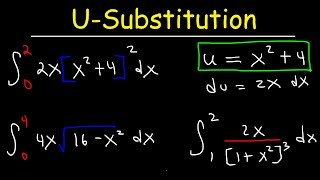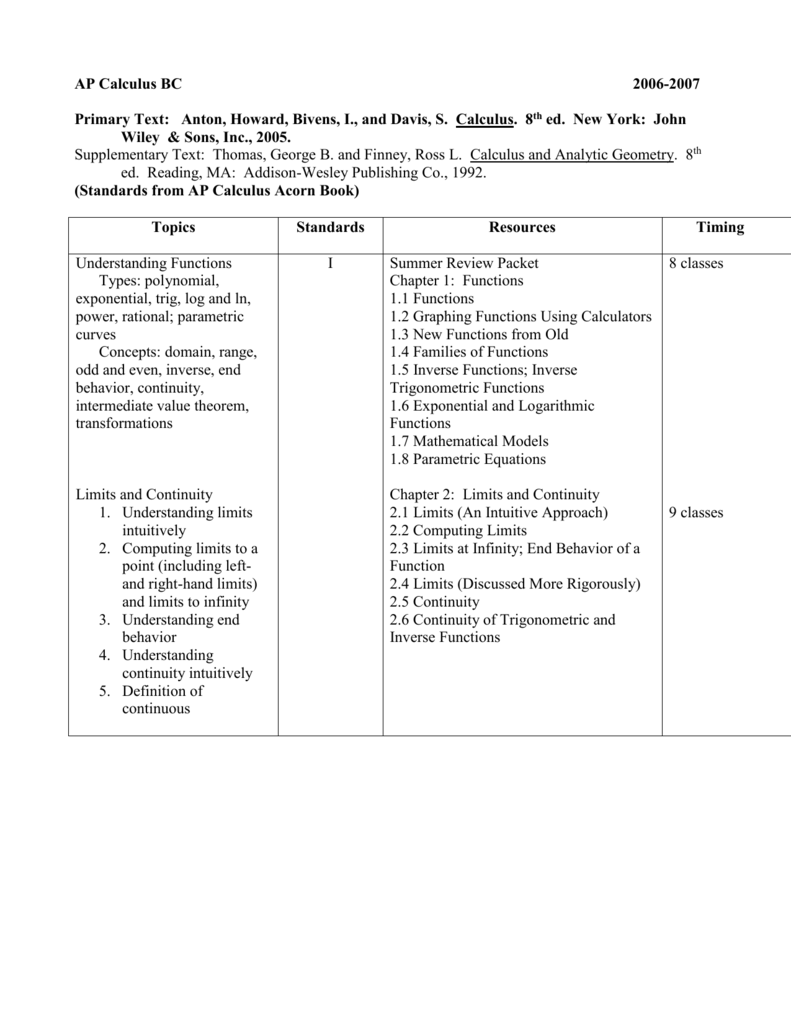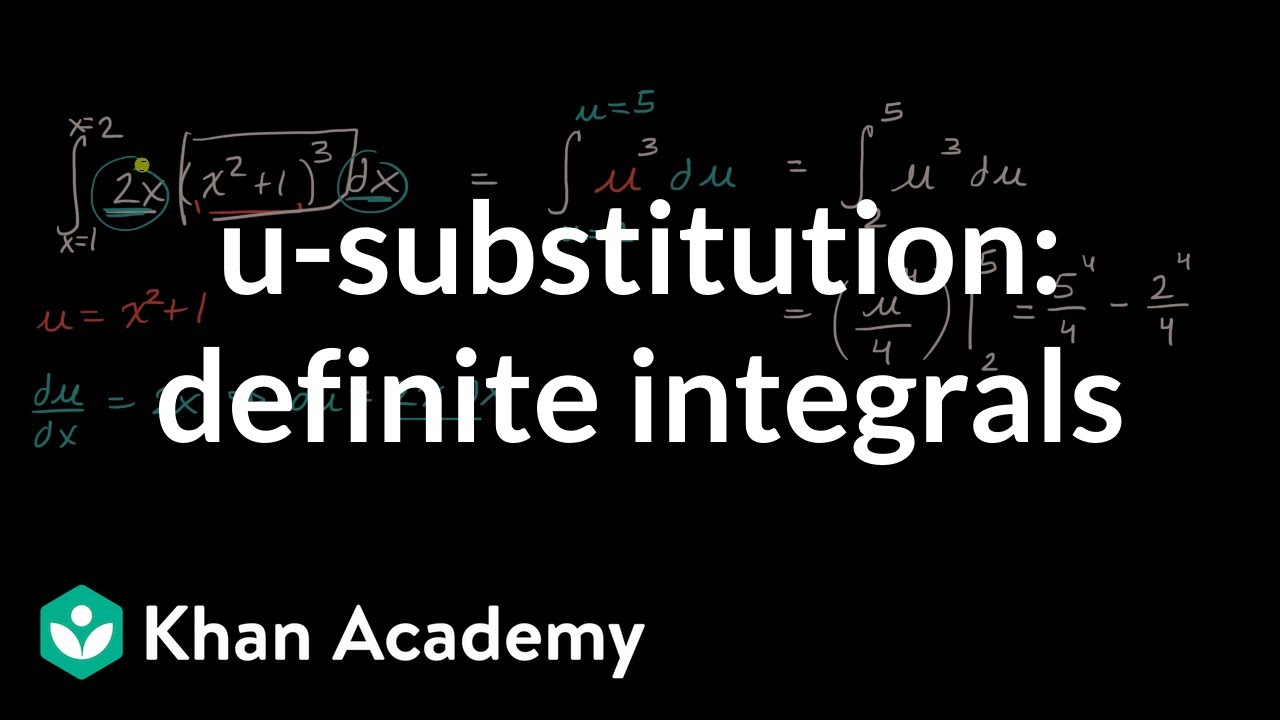# 10.3 Usubstitution Definite Integralsap Calculus

Let's take a look at an integral problem. Consider the indefinite integral of x times the square root of x squared minus 9. Though you'd probably solve this using the method of substitution, you'd probably think substitute for the x squared minus 9 part. But how would you solve the, the definite integral from 3 to 5. Same integram? It turns out that you can use the method os substitution for a definite integral as well. I want to show you how to do that.
So let's copy this down, the integral from 3 to 5 x times the square root of x squared minus 9. So we'll substitute for the x squared minus 9. w=x squared minus 9. And dw will equal 2x dx. Now I don't have a 2x dx but I do have an x dx. So I can just divide both sides by 2 and get one half dw equals x dx. That'll take care of everything inside the integral but it does not take care of the limits. These limits are x values. When I change this integral over to w, I really should change the limits to w values as well. And you can do that using this substitution. Right? x=3 for example this bottom limit, if you plug 3 in here you get 9, minus 9 is 0. w=0. So the 3 is going to turn into a 0 and x=5. If you plug that in here you get 25-9. w is going to be 16. So my new integral's going to be the integral from 0 to 16. The square root of x squared minus 9, that will be root w the x dx, one half dw. So I'll pull the one half outside. Integral from 0 to 16 w to the one half dw. This is one half and now I need an anti-derivative for w to the one half. I raise the exponent by 1 to w to the 3 halves and divide by 3 halves which is the same as multiplying by 2 thirds. So this is 2 thirds w to the 3 halves. And I want to evaluate this from 0 to 16. Now one half times 2 thirds is one third. So it's one third 16 to the 3 halves minus one third 0 to 3 halves. Now, of course this is going be 0 but what's 16 to the 3 halves. Whenever I have a fractional exponent depends on what the input is. As to whether I want a cube first or take the square root first but this number is a perfect square. So I'm going to take the square root first.
The square root is 4 then raised to the third power is 64. So this is 64 thirds. And that's my answer. So the new trick here is switching over the limits of integration using your substitution. Remember this formula tells you how. These are x values. Plug them in here and you can get the corresponding w values.

The Substitution Method for Integration. Integration by substitution is one of the many methods for evaluation of integrals in calculus. Other methods of integration include the use of integration. 5 Integration 5.2 The Definite Integral 5.4 The Fundamental Theorem of Calculus 5.3 Riemann Sums In the previous section we defined the definite integral of a function on a, b to be the signed area between the curve and the x -axis.

### Definite Integral Example on the TI-89: Raw Transcript## 10.3 U-substitution Definite Integralsap Calculus Algebra## 10.3 U-substitution Definite Integralsap Calculus Solver## 10.3 U-substitution Definite Integralsap Calculus Integrals

This is a video on u substitution and this one is about a definite integral
where when you actually compute the integral you are going to compute it over a range
with a lower limit and a upper limit so anytime you have a problem like I’m going
to show you here that’s the way you use my programs
you go to u substitution let’s get started here
we’re going to press 2nd alpha and put i n d e x
into the entry line of the calculator then press alpha and put the eight and the
open and closed parenthesis press enter and you’re into my menu
you have many, many things on here notice graphing by hand
and all kinds of things that are perfectly done
and that’s what we’re interested in is passing calculus
and never to do it again so anyways you can scroll up or down
to u substituion this is all alphebetical and this case you
want u substitution so we might press the upper cursor to go up
and to the bottom menu, which is u substituion
notice you have trig d dx integrals for trig
half angle formulas all kinds of things you need for calculus
one for sure and then I have other programs for calculus
two and three so if I press enter we’re into u substitution
and generally you press alpha and put your function in here
you have to press alpha first and then put the function in
but I’ve already done that to speed up the video so i’m going to
I always show you, this is the function we’re doing
I haven’t put the limits in yet so you have to recognize that
you are going to do the integral first and then at the end I ask you if you want
to do the range for the area or limits
now, I always show you what you’ve entered so you can change it if you want
I say it’s ok and we’re going to evaluate this integral
here now you’ll notice that the derivative of three
x to the five is really fifteen x to the four
and here’s an x to the four so if you didn’t have my calculator
that is what you are really looking at everytime you look at an integral
you say well is the inside, can you do the derivative
to make it equal to the outside somehow and
but I do that for you in my programs we always rewrite it
anytime you have a number or constant inside the integral
you take it outside the integral like I’ve done here in my programs
and then you evaluate the integral inside but also when you rewrite it you need to take
this x to the four and put it over here by the
dx and then you choose the u which is the three
x to the five plus two and the derivative of that is fifteen x to
the four anytime you have a number here before the
x you need to transpose it to the other side
by division so you are going, which I’ve done here now
du divided by 15 equals x to the four dx you’ll notice that this equals what we re-wrote
in the beginning here and so we know that that is a u substitution
problem and I ask you that too
because I need to check that before and so I ask you if x to the four equals x
to the four if it says yes, if they’r equal you say yes
of course if it’s not equal you say no if you say no then you are into log rhythms
because in integrals you can’t do integrals with a times sign or
division sign you need to seperate it into plus and minus
parts so that you can integrate it
and that’s what log rhythms do for instance in times you are taking a log
of one factor plus the log of the other factor in division you are taking the log of one
factor minus the other.
so anyway they are equal so we’re going to get on with this
you write all of this on your paper and write it kind of sloppy
students and people that really know this stuff write sloppy
that’s been my experience, including professors they scribble because they want to make everyone
know that they are genius’s at this stuff.
so you do the same don’t write it clean like this write it sloppy
and uhm, here’s the answer after you do all the u
you’ll notice the du divided by fifteen you need to bring that to the outside of integral
which I do one fifteenth times six here
multiply that together to get two fifths and then you do the integral of u to the 6
which you add one to the six and get seven and divide by seven
u seven divided by seven well then you have to do the computation there
seven times five is thirty five so you have two over thirty five
now you substitute back in the u which is thirty five plus two
and you have your answer plus c now the really tricky part here
I ask you if you want to evaluate the range you can press one here and do it
let’s do the limit which I’ve put in there as number one
for the lower range or limit and number two for the upper limit
and so here’s the integral that I showed you at the
beginning of the video. which is called a definite integral
where you find the area under the curve and again if there is a mistake in adding
the limits I ask you that so you can change them if you
want now you’re going to substitute
if x equals one then u equals
remember we’ve decided that u equals this and then you add the one in there and mulitiply
it out to get five ok?
if x equals two you put this exactly on you paper, here’s
what your doing u equls three x two
and that equals ninety eight so you have u substituted here and you have
the upper limit ninety eight and five notice you can’t use one and two for the limit
because you’ve changed it to the u system, so
and then with u equals ninety eight the upper limit
you have to go back to the original function and put it in here the original integral that
you’ve found and that equals four point nine six times
e to the twelve and then when, the lower limit is five so
you are adding that into the original u funtion to get four point four three time e to the
3 you substract the upper limit
the lower limit from the upper limit here’s the area under the curve
four point nine six times e to the twelve square units
uhm, notice how fabulous these programs are and how they will help you so much
even in learning this stuff let alone passing tests
or for homework so you can buy my programs at
every step calculus dot com and enjoy my programs
and pass calculus